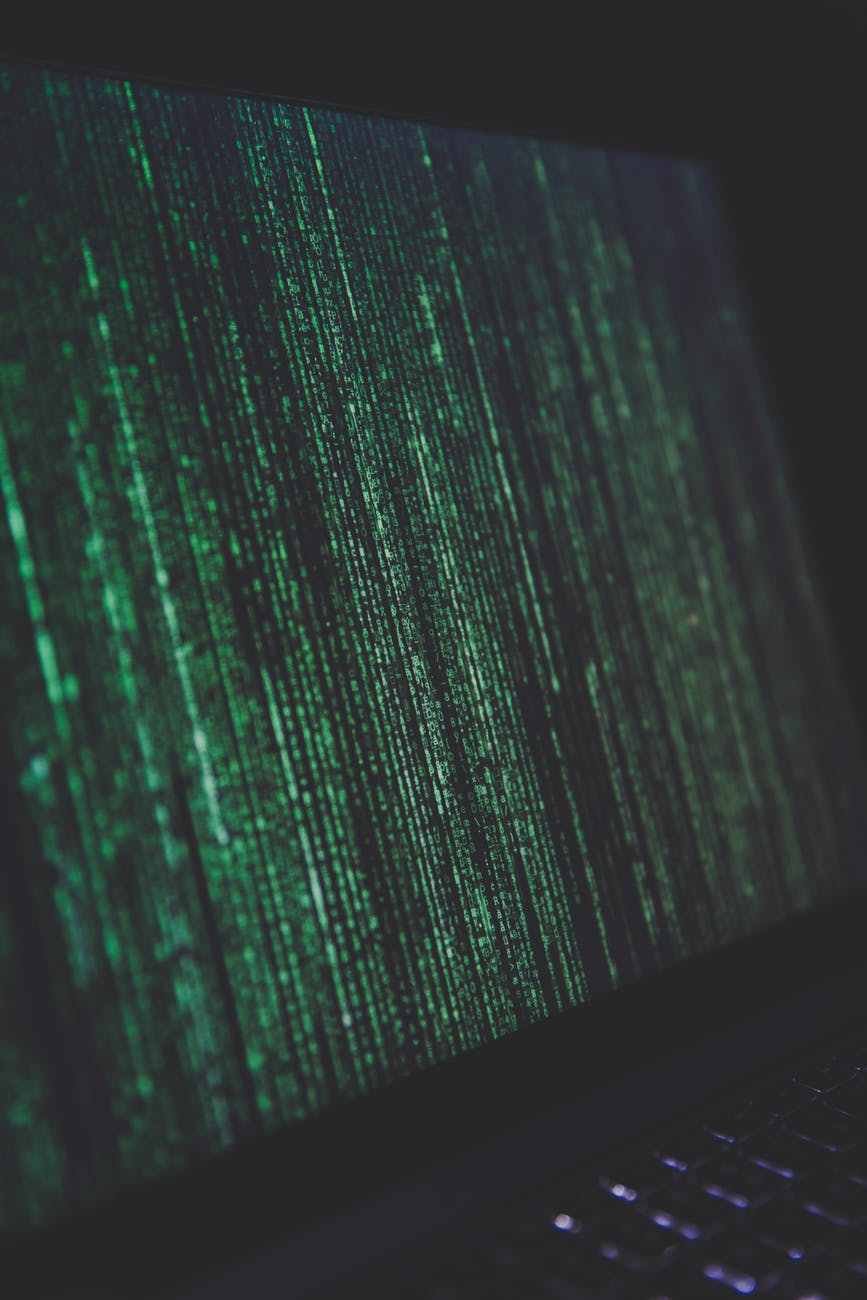# New theory streamlines development of quantum algorithms

Google claimed it was the first to demonstrate a quantum computer performing a calculation beyond the abilities of today’s most powerful supercomputers.

But most of the time, creating a quantum algorithm that stands a chance at beating a classical computer is an accidental process, Purdue University scientists say. To bring more guidance to this process and make it less arbitrary, these scientists developed a new theory that may eventually lead to more systematic design of quantum algorithms.

The new theory, described in a paper published in the journal Advanced Quantum Technologies, is the first known attempt to determine which quantum states can be created and processed with an acceptable number of quantum gates to outperform a classical algorithm.

Physicists refer to this concept of having the right number of gates to control each state as “complexity.” Since the complexity of a quantum algorithm is closely related to the complexity of quantum states involved in the algorithm, the theory could therefore bring order to the search for quantum algorithms by characterizing which quantum states meet that complexity criteria.

An algorithm is a sequence of steps to perform a calculation. The algorithm is usually implemented on a circuit.

In classical computers, circuits have gates that switch bits to either a 0 or 1 state. A quantum computer instead relies on computational units called “qubits” that store 0 and 1 states simultaneously in superposition, allowing more information to be processed.

What would make a quantum computer faster than a classical computer is simpler information processing, characterized by the enormous reduction in the number of quantum gates in a quantum circuit compared with a classical circuit.

In classical computers the number of gates in circuits increases exponentially with respect to the size of the problem of interest. This exponential model grows so astonishingly fast that it becomes physically impossible to handle for even a moderately sized problem of interest.

“For example, even a small protein molecule may contain hundreds of electrons. If each electron can only take two forms, then to simulate 300 electrons would require 2300 classical states, which is more than the number of all the atoms in the universe,” said Sabre Kais, a professor in Purdue’s Department of Chemistry and member of the Purdue Quantum Science and Engineering Institute.

For quantum computers, there is a way for quantum gates to scale up “polynomially” – rather than just exponentially like a classical computer – with the size of the problem (like the number of electrons in the last example). “Polynomial” means that there would be drastically fewer steps (gates) needed to process the same amount of information, making a quantum algorithm superior to a classical algorithm.

Researchers so far haven’t had a good way to identify which quantum states could satisfy this condition of polynomial complexity.

“There is a very large search space for finding the states and sequence of gates that match up in complexity to create a useful quantum algorithm capable of performing calculations faster than a classical algorithm,” said Kais, whose research group is developing quantum algorithms and quantum machine learning methods.

Kais and Zixuan Hu, a Purdue postdoctoral associate, used the new theory to identify a large group of quantum states with polynomial complexity. They also showed that these states may share a coefficient feature that could be used to better identify them when designing a quantum algorithm.

“Given any quantum state, we are now able to design an efficient coefficient sampling procedure to determine if it belongs to the class or not,” Hu said.

IMAGE SOURCE: Creative Commons